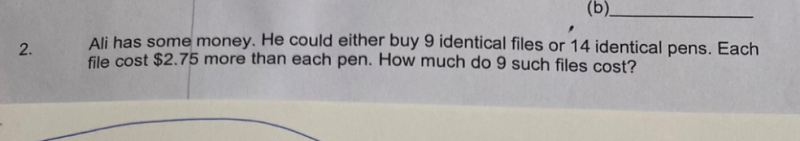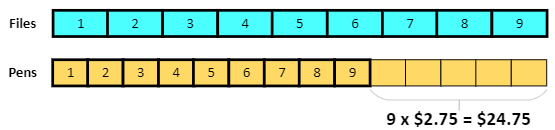# QuestionHi there!

kindly help with this question without algebra.```1 File  - 1 Pen  = 2.75
9 Files - 9 Pens = 9 x 2.75 = 24.75
If we buy 9 Pens, we have \$24.75 left.
That amount can buy 14 - 9 = 5 Pens.
So 1 Pen = 24.75/5 = \$4.95.
Thus 9 Files = 14 Pens = 14 x 4.95 = \$69.30.

Check:
1 File = 69.30/9 = \$7.70
1 File - 1 Pen = 7.70 - 4.95 = \$2.75```
0 Replies 2 Likes ✔Accepted Answer